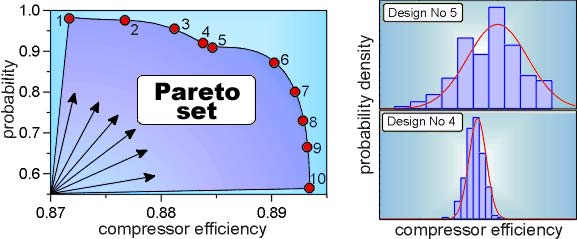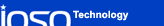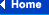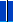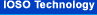### Robust design optimization and robust optimal control

##### Introduction

Practical application of the numerical optimization results is difficult because of the fact that any complex technical system is a stochastic system and the characteristics of this system have probabilistic nature. We would like to emphasize  that, speaking about the stochastic properties of a technical system within the frame of optimization tasks, we imply that the important parameters of any system are stochastically spread. Normally it occurs during the production stage despite of the up-to-date level of modern technology. Random deviations of the system parameters lead to a random change in system efficiency.

An efficiency extreme value, obtained during the optimization problem while solving in traditional (deterministic) approach, is simply a maximum attainable value and can be considered as just conventional optimum from the point of view of its practical realization. Thus, one can consider two different types of optimization criteria. One of them is an ideal efficiency which can be achieved under the conditions of absolutely precise practical replication of the system parameters under consideration. Other optimization criteria are of probabilistic nature. For example: mathematical expectation of the efficiency; the total probability of assuring preset constraints; variance of the efficiency and so on

It is evident that the extreme of the one of these criteria doesn't guarantee the assurance of the high level of another one. Even more, these criteria may contradict to each other. Thus, in this case we have a multicriteria optimization problem.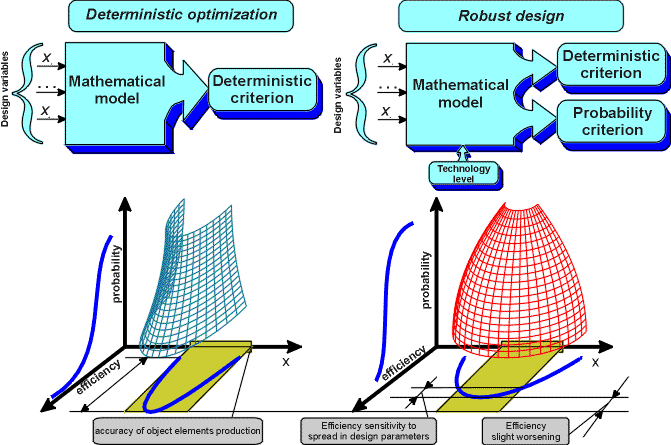##### Our concept

Our concept of robust design optimization and robust optimal control allows to determine the optimal practical solution that could be implemented with the high probability for the given technology level of the production plants. Many modern probabilistic approaches either employ the estimation of probabilistic efficiency criteria only at the stage of the analysis of obtaining deterministic solution, or use significantly simplified assessments of probabilistic criteria during optimization process. The distinctive feature of our approach is that during robust design optimization we solve the optimization problem involving direct stochastic formulation, where the estimation of probabilistic criteria is accomplished at each iteration. This procedure reliably produces fully robust optimal solution. High efficiency of the robust design optimization is provided by the capabilities of IOSO algorithms to solve stochastic optimization problems with large level of noise.

Our robust design optimization concept provides considerable (several orders of magnitude) reduction in cost and time during the development of the new highly efficient systems. This concept also considerably (several times) reduces risk associated with new technical solutions.

For example, during optimal calibration of the automotive engine the development time was reduced by 5 times, during development of the new axial compressor more than 200 times. The most important feature of IOSO technology is the ability to solve robust design optimization problems with the large number of variables (hundreds) and efficiency criteria (dozens).

##### Real-life example
MULTICRITERIA ROBUST DESIGN OF THE MULTISTAGE AXIAL FLOW COMPRESSOR
##### Problem features: 140 design variables, 2 criteria.